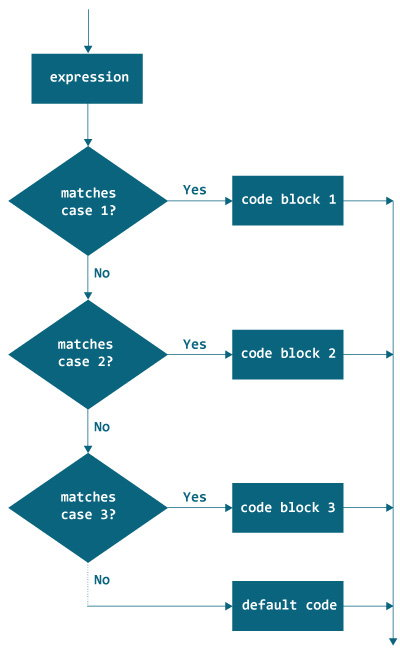# Java switch Statement

In this article, you will learn to use switch statement to control the flow of your program’s execution

In Java, the if..else..if ladder executes a block of code among many blocks. The `switch` statement can a substitute for long `if..else..if` ladders which generally makes your code more readable.

The syntax of switch statement is:

```switch (variable/expression) {
case value1:
// statements
break;
case value2:
// statements
break;
.. .. ...
.. .. ...
default:
// statements
}```

The `switch` statement evaluates it's expression (mostly variable) and compares with values(can be expression) of each `case` label.

The `switch` statement executes all statements of the matching `case` label.

Suppose, the `variable/expression` is equal to value2. In this case, all statements of that matching case is executed.

Notice, the use of `break` statement. This statement terminates the execution of `switch` statement. The break statements are important because if they are not used, all statements after the matching `case` label are executed in sequence until the end of `switch` statement.

## Flowchart of switch StatementIt's also important to note that `switch` statement in Java only works with:

• Primitive data types: `byte`, `short`, `char` and `int`
• Enumerated types (Java enums)
• `String` class
• a few classes that wrap primitive types: `Character`, `Byte`, `Short`, and `Integer`.

## Example 1: Java switch statement

``````class Day {
public static void main(String[] args) {

int week = 4;
String day;

switch (week) {
case 1:
day = "Sunday";
break;
case 2:
day = "Monday";
break;
case 3:
day = "Tuesday";
break;
case 4:
day = "Wednesday";
break;
case 5:
day = "Thursday";
break;
case 6:
day = "Friday";
break;
case 7:
day = "Saturday";
break;
default:
day = "Invalid day";
break;
}
System.out.println(day);
}
}
``````

When you run the program, the output will be:

`Wednesday`

## Example 2: Java switch statement

The program below takes three inputs from the user: operator and 2 numbers. It performs calculation based on numbers and operator entered. Then the result is displayed on the screen.

We have used `Scanner` object to take input from the user. To learn more, visit Java Basic Input.

``````import java.util.Scanner;

class Calculator {
public static void main(String[] args) {

char operator;
Double number1, number2, result;

Scanner scanner = new Scanner(System.in);
System.out.print("Enter operator (either +, -, * or /): ");
operator = scanner.next().charAt(0);
System.out.print("Enter number1 and number2 respectively: ");
number1 = scanner.nextDouble();
number2 = scanner.nextDouble();

switch (operator) {
case '+':
result = number1 + number2;
System.out.print(number1 + "+" + number2 + " = " + result);
break;

case '-':
result = number1 - number2;
System.out.print(number1 + "-" + number2 + " = " + result);
break;

case '*':
result = number1 * number2;
System.out.print(number1 + "*" + number2 + " = " + result);
break;

case '/':
result = number1 / number2;
System.out.print(number1 + "/" + number2 + " = " + result);
break;

default:
System.out.println("Invalid operator!");
break;
}
}
}
``````

When you run the program, the output will be:

```Enter operator (either +, -, * or /): *
Enter number1 and number2 respectively: 1.4
-5.3
1.4*-5.3 = -7.419999999999999```